﻿

###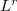$L^r$ inequalities for the derivative of a polynomial

#### Abstract

Let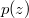$p(z)$ be a polynomial of degree$n$ having no zero in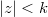$|z|< k$,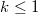$k\leq 1$, then Govil [Proc. Nat. Acad. Sci.,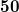$\textbf{50}$, (1980), 50-52] proved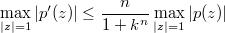$\max\limits_{|z|=1}|p'(z)|\leq \dfrac{n}{1+k^{n}}\max\limits_{|z|=1}|p(z)|$,

provided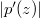$|p'(z)|$ and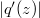$|q'(z)|$ attain their maxima at the same point on the circle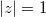$|z|=1$, where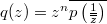$\label{A}q(z)=z^{n}\overline{p\left(\frac{1}{\overline{z}}\right)}$.

In this paper, we not only obtain an integral mean inequality for the above inequality but also extend an improved version of it into$L^{r}$ norm.

DOI Code: 10.1285/i15900932v41n2p19

Keywords: Inequalities; Polynomials; Zeros; Maximum modulus; Lr norm

Full Text: PDF